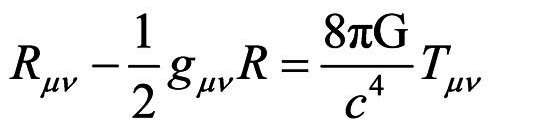## Have the Einstein field equations changed their meaning?In his short essay, “How Great Equations Survive,” Nobel laureate physicist Steven Weinberg argues that though equations survive through scientific change, they are reinterpreted in light of the developments of theory. “The equations of General Relativity,” he argues, “have undergone a similar reinterpretation.” Weinberg devotes attention to the point that “the equations” are of the type known as “second order differential equations." This means "that the equations were assumed by Einstein to involve only rates of change of the fields (first derivatives) and rates of change of rates of change (second derivatives) but not rates of higher order.” This he sees as something of a reasonable idealization. “I don’t know,” he writes, “any place where Einstein explains the motivation for this assumption.”“Today,” Weinberg continues, “General Relativity is widely (though not universally regarded as another effective field theory, useful only for distances much larger than about 10 (to the -33rd) centimeters, and particle energies much less than an energy equivalent to the mass of 10 (to the 19th) protons. No one today would (or at least no one should) take seriously any consequence of General Relativity for shorter distances or larger energies.”